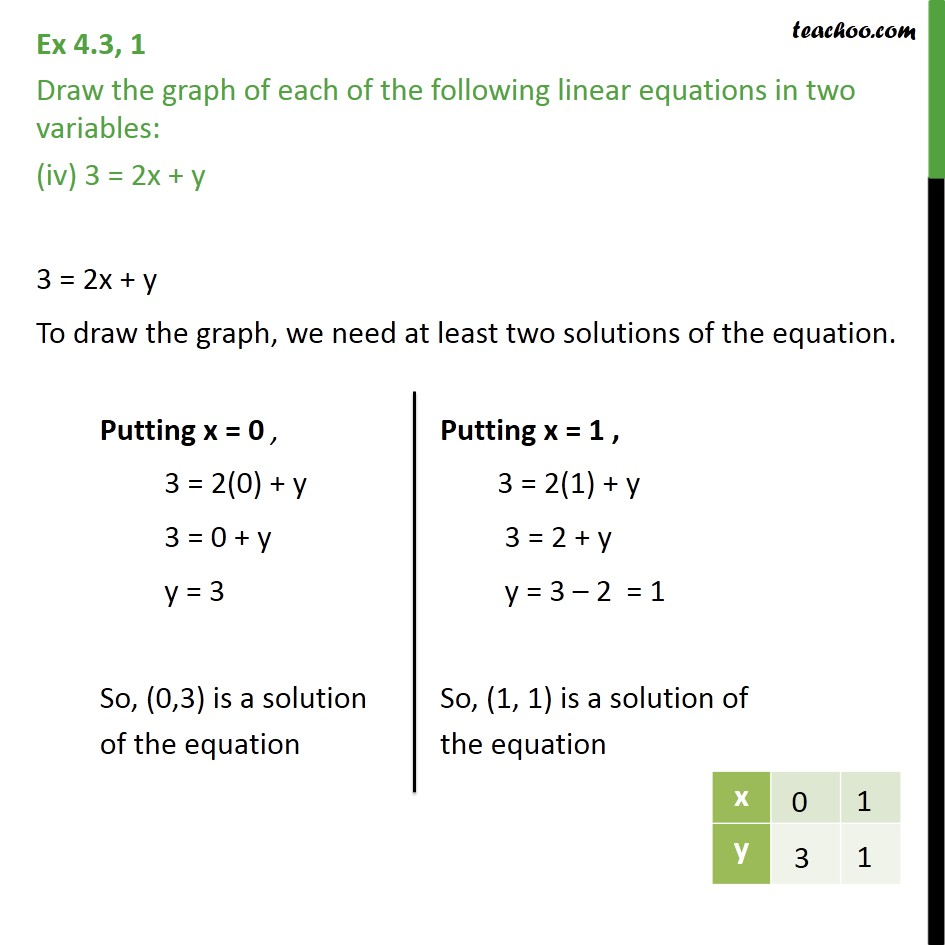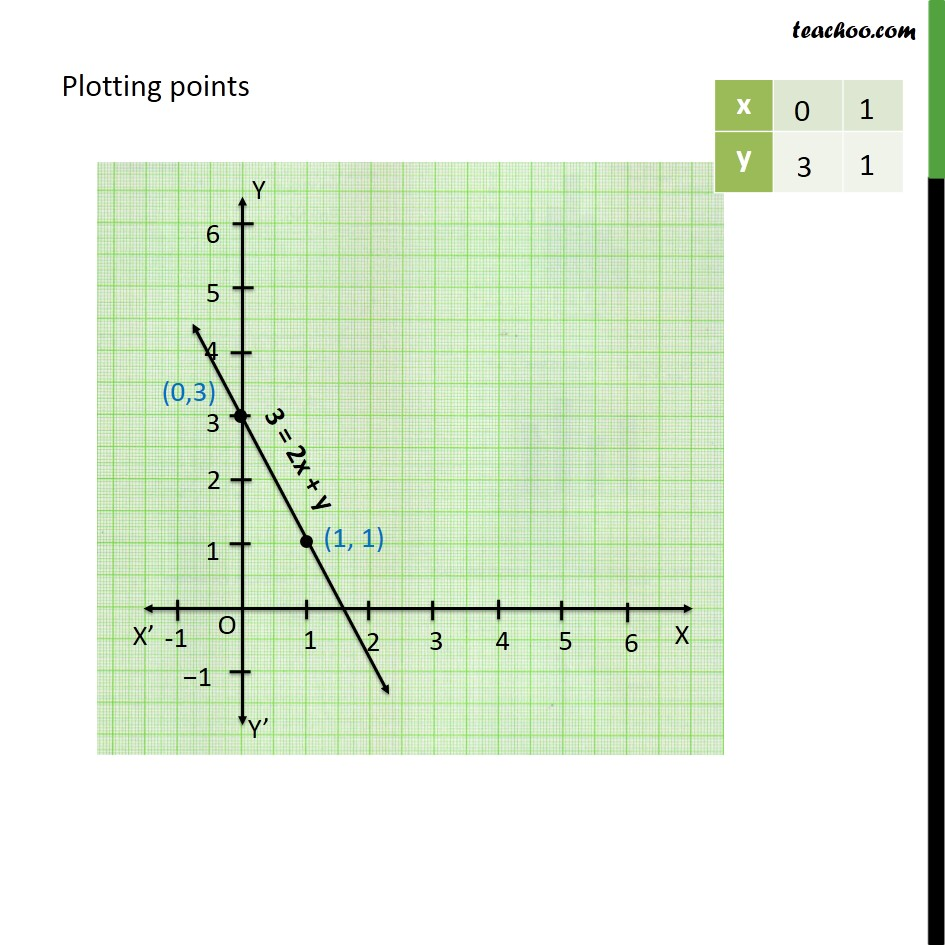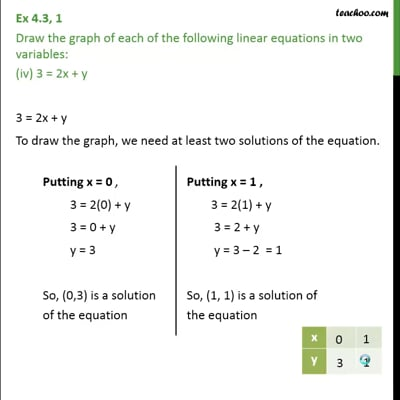Ex 4.3

Chapter 4 Class 9 Linear Equations in Two Variables
Serial order wiseThis video is only available for Teachoo black users

Maths Crash Course - Live lectures + all videos + Real time Doubt solving!

### Transcript

Ex 4.3, 1 Draw the graph of each of the following linear equations in two variables: (iv) 3 = 2x + y 3 = 2x + y To draw the graph, we need at least two solutions of the equation. Plotting points